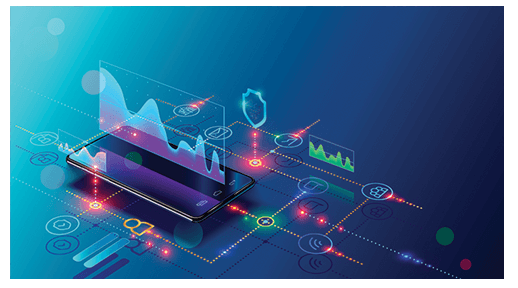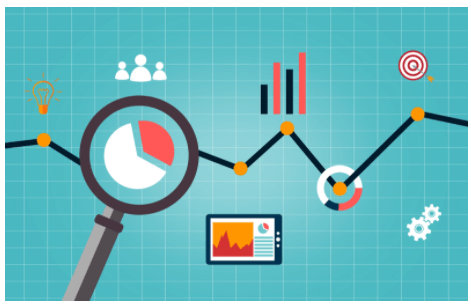# An Introduction to Key Data Science Concepts

Data science is a field of study that integrates domain expertise, programming skills, and understanding of math and statistics to extract useful insights from data. As a result, these systems produce insights that analysts and business users may employ to create tangible business value. It involves processing large datasets containing structured and unstructured data and assists businesses in better understanding and enhancing their processes, resulting in efficient time management as well as reduction of costs.

Social media, phone data, e-commerce sites, healthcare surveys, internet searches, and other fields and platforms are used to collect data. As the amount of data available grows, a new field of research known as Big Data – or exceptionally huge data sets – emerges; this can aid in the development of better operational tools in a variety of fields.

Your Amazon purchases, Facebook feed, and even the face recognition required to sign in to your phone all include data. Models that automatically self-improve, recognizing and learning from their failures, are created by expert data scientists working in machine learning and AI.

Data is swiftly becoming the most valuable commodity. Organizations all around the world are focusing on approaches to organize and use data in order to achieve their strategic goals. According to the World Economic Forum’s Future of Work Report 2020, by 2025, the most in-demand and fastest-growing employment will be that of a Data Scientist.

In this article, we shall discuss some of the key data science concepts.## Key data science concepts:

### 1.Descriptive Statistics

Descriptive statistics is the name given to a concept of data analysis that helps to describe, show, or summarize data in a comprehensible way so that patterns might emerge. Descriptive statistics do not allow us to draw conclusions beyond the data we’ve examined or to form conclusions about any hypotheses we’ve proposed. They’re just a way of describing our data in a relatively simpler way.

It differs from inferential statistics in that it uses charts to assist and visualize data in a meaningful way. Inferential statistics, on the other hand, help in finding insights from data analysis.

### 2.Probability

Randomness and uncertainty are inevitable in the world; thus, understanding and knowing the odds of certain events can be pretty beneficial. Probability theory is the mathematical foundation of statistical methodology, and it is vital for data scientists to analyze data that is affected by chance. Depending on the type of event, different types of probability exist.

Two or more events are independent if the occurrence of one event doesn’t have an effect on the probability of the other. The likelihood of occurrence of any event that has a link with another event is known as conditional probability.

### 3.Central Tendency

•     Mean- It is the sum of all values divided by the total number of values.
•     Median- It’s the middle number in a systematically arranged data set.
•     Mode- It’s the value that occurs the most frequently in a dataset.

Skewness is a measure of a distribution’s symmetry. The mode of a distribution is its highest point. The mode denotes the x-axis response value that occurs with the highest probability. If the tail on one side of the mode is longer than the tail on the other, the distribution is skewed: it is asymmetrical.

Kurtosis is a statistical measure about how much a distribution’s tails diverge from the tails of a normal distribution. In other words, kurtosis determines whether a distribution’s tails contain extreme values.

### 4.Dimensionality Reduction

The process of lowering the number of dimensions of features in a dataset is known as dimensionality reduction or variable reduction techniques. The transformation of data from a high-dimensional space to a low-dimensional space is in such a way that the low-dimensional representation retains some of the original data’s meaningful properties, ideally close to its intrinsic dimension. The dimensionality reduction concept has many potential advantages, including less redundancy, faster computing, and fewer data to store.

### 5.Hypothesis Testing

Hypothesis testing is a statistical procedure in which an analyst tests a hypothesis about a population parameter. The analyst’s approach is determined by the type of the data and the purpose of the analysis.

A null hypothesis is a statement that claims there is no relationship between two measurable events. It’s an assumption, likely based on domain expertise.

The alternative hypothesis in statistical hypothesis testing claims something is happening and that a new theory is preferred over an old one. The Alternate hypothesis is a statement that contradicts the Null hypothesis.

### 6.Test of Significance

Subjective interpretations cannot be relied upon by researchers. To make a claim, researchers must gather statistical evidence, which is done through a statistical significance test. It helps to test the validity of the cited Hypothesis.

•   The p-value is used to describe the level of statistical significance. You will calculate a probability (the p-value) of observing your sample results or more extremes, given that the null hypothesis is true, depending on the statistical test you have chosen.
•   When the variances are known, and the sample size is large, the Z-test is used to see if the two population means are different. In the Z-test, the z-statistic follows a normal distribution. A z-statistic, often known as a z-score, is a numerical representation of the z-test result.
•   A t-test is an inferential statistic that is used to see if there is a significant difference in the means of two groups that may be related in some way. Three fundamental data values are required to calculate a t-test. They include the mean difference (difference between the mean values in each data set), the standard deviation of each group, as well as the number of data values in each group.
See also  Targeting IIT? Here is why you will need help from a coaching

### 7.Sampling

Sampling is a part of statistics that includes collecting, analyzing, and interpreting data gathered from a random sample set of the population.

Under-sampling is the process of removing redundant data, while oversampling is the process of replicating a naturally occurring data sample.

### Conclusion-

Data Scientists and Business Analysts use statistics to process complex problems in the real world so that they may look for relevant trends and changes in data. In other words, statistics can be used to derive valuable insights from data through mathematical computations. To analyze raw data, develop a Statistical Model, and infer or anticipate the result, several statistical functions, principles, and algorithms are used.

### FAQs

#### 1.What are the most important skills for a data scientist to have?

Some of the most important skills for data scientists include Fundamentals of Data Science, Statistics, Programming knowledge, Data Manipulation and Analysis, Data Visualization, Machine Learning, Deep Learning, Big Data, Software Engineering, Model Deployment, Structured Thinking, and Curiosity.

#### 2.Why are math skills necessary for data scientists?

Data science’s growth necessitates a boost in executive statistics and math skills. Correlation, causation, and how to statistically evaluate hypotheses are some of the essential concepts expected of a data scientist and business analyst.